# Congruence and Rigid Transformations

## Intro: What do we mean by corresponding parts

Please watch this video, Corresponding Parts before starting the assignment, it explains what corresponding parts are.

## a.

Translate quadrilateral ABCD from point C to point V. *Select the vector tool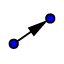, and draw a vector starting at point C and ending at point V. Then, select the translate by vector tool, click on quadrilateral ABCD, and then click on the vector. *If you need help, watch this video on how to do a Translation

## b.

After you have translated quadrilateral ABCD, select the angle tool, and measure all four interior angles on the pre-image and image. What do you notice about every pair of corresponding angles? *Video help on how to Measure Angles.

## c.

Now, grab the distance measuring tool,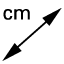, and measure all four side lengths on both the pre-image and the image. What do you notice about every pair of corresponding sides? *Video help on how to Measure Side Lengths.

## a. Reflect triangle DEF over line m

Select the reflect about line tool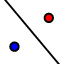, click on triangle DEF, then select line m. *Video help on how to do a Reflection.

## b.

Just like in question 1, measure all interior angles and all side-lengths on both the pre-image (triangle DEF) and the image (triangle D'E'F'). What do you notice about pairs of corresponding sides and angles?

## a. Rotate triangle JKL 180 degrees using V as the center of rotation

*Select the rotate around point tool, click on triangle JKL, then click on point V. Type in 180 degrees in the menu (counterclockwise or clockwise does not matter) *Video help on how to do a Rotation.

## b.

Just like in question 2, measure all pairs of corresponding sides and interior angles. Based on our work in questions 1-3, what can we conclude about corresponding sides and angles under one or more rigid transformations?

## Summary of questions 1-3

Corresponding Parts: Corresponding sides and angles are a pair of matching angles or sides that are in the same spot in two different shapes. The shapes must either be similar or congruent.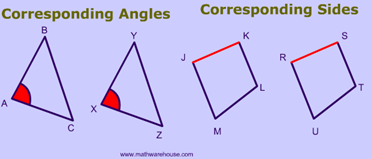rigid transformation is a transformation for which all pairs of corresponding lengths and angle measures in the original figure and its image are equal. Translations, rotations, and reflections have this property, so they are rigid transformations.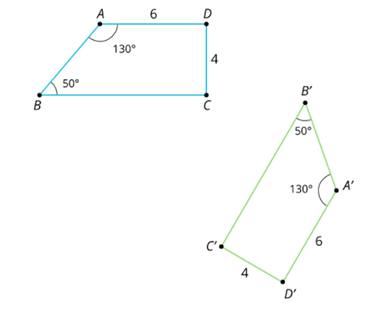## Guide for questions 4-5

Please click on the following video links before you work on questions 4-5. Example A, helps with question 4. Example B, helps with question 5. Really pay attention to this one.

## 4.

Determine if trapezoid ABCD is congruent to Trapezoid QRST.  If they are congruent, prove it using a sequence of rigid transformations. Illustrate your steps with GeoGebra. If they are not congruent, prove it by talking about differences in corresponding sides and angles.

## 5.

Determine if triangle ABC is congruent to Triangle DEF. If they are congruent, prove it using a sequence of rigid transformations.  Illustrate your steps with GeoGebra. If they are not congruent, prove it by talking about differences in corresponding sides and angles.